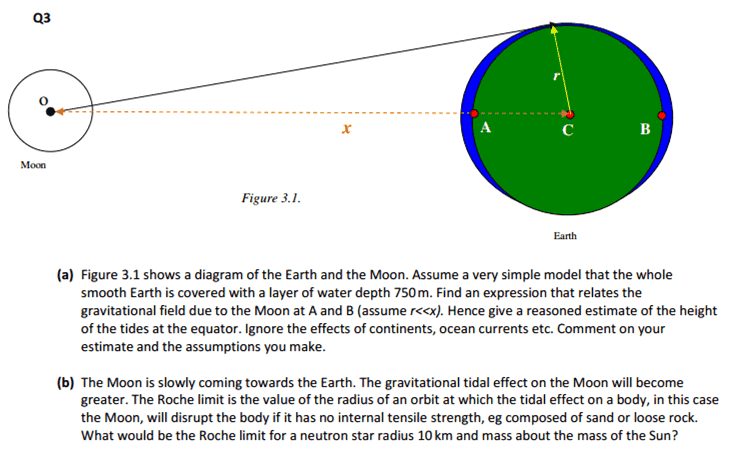# Differential gravitational force causing tides model?

## Homework Statement## The Attempt at a Solution

For a), I initially tried to consider the component of the gravitational force of the moon acting normally to the earth's surface. This would be F=F_0 cos(theta) where theta is the angle between a horizontal line going through A and B and the point on the earth's surface in question (giving zero at the poles and maximum at the equator). I know that , due to the effect of the Earth actually undergoing circular motion and 'free-falling' due to the moon's gravitational pull, the force at each point on the side of the Earth facing the moon will be (approximately) mirrored by the opposite side of the earth since r<<x. But now I am stuck. I wanted to deal with forces rather than equipotentials, as it seems like the question wants you to just deal with the forces. I was considering the fact that the effect is actually spherically symmetrical when looking onto the face of the Earth from the moon, but II don't know what to do with this to find the heights? I could find the total volume of water on the Earth by doing surface area of earth x 750, but I still don't see how II could use this?

mfb
Mentor
as it seems like the question wants you to just deal with the forces
I don't see why. Potentials are fine.

I don't see why. Potentials are fine.

Help! I cannot figure out how to get the equation with the equipotential. Would I have to consider the earth's rotation? And how to I create a potential due to the moon?

mfb
Mentor
And how to I create a potential due to the moon?
In the same way you do for earth, with Newton's law of gravity.
Would I have to consider the earth's rotation?
Not the 24h-rotation, but the motion around the center of mass of the earth/moon system is relevant to get the second tidal wave at the opposite side.